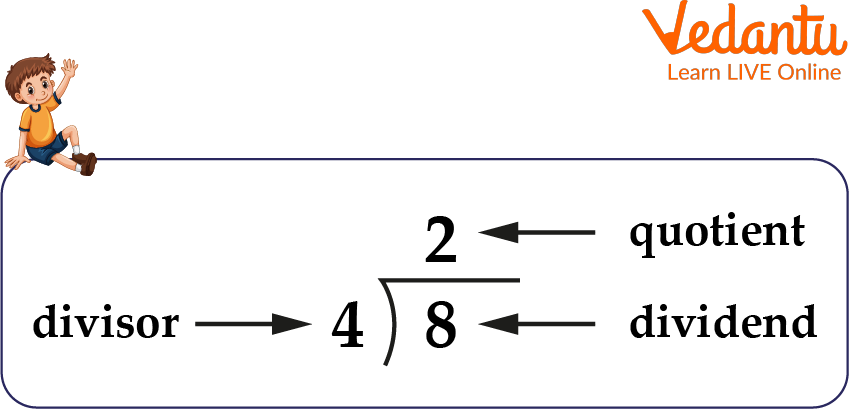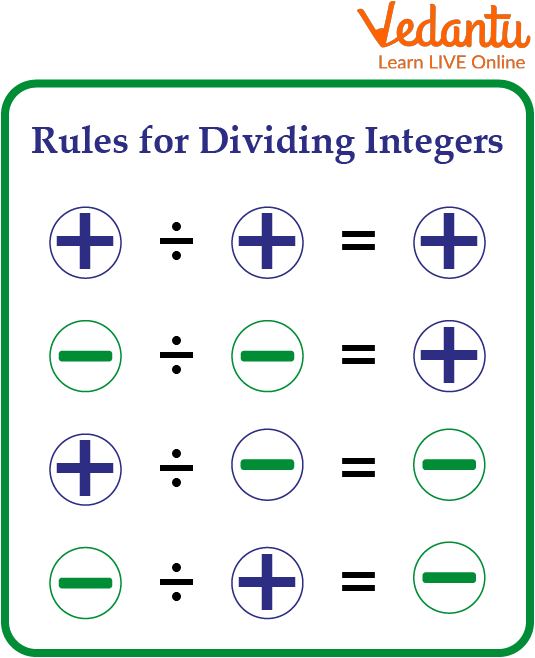Courses
Courses for Kids
Free study material
Free LIVE classes
More

# Rules of Division of IntegersLIVE
Join Vedantu’s FREE Mastercalss

## A Brief Explanation of the Division of Integers

Mathematicians use addition, subtraction, division, and multiplication to perform arithmetic operations on all various areas of real numbers, including natural numbers, integers, and rational numbers. A division of integers is a process of dividing integers. When an integer is divided, it is usually evenly divided into groups or divided into a specified number of groups. Here, in this article, students will learn about the definition of dividing integers, and about what is the rule for dividing integers. Some examples are also discussed by applying the learning.

## Division of Integers

Integer division works by dividing an integer value into equal parts. The division consists of three parts. The value that has been divided into equal groups is called the dividend. The value that divides into equal groups is known as the divisor. Additionally, the answer obtained is called the quotient, which clarifies how many components are divided into equal groups.Division of Integers

## What are the Rules for Dividing Integers?

Here we have listed some rules that are to follow while dividing integers:Rules of Division of Integers

Rule 1: To divide two numbers with similar signs, we divide their values and add a plus sign to the quotient.

Here, we divide two positive integers that have a positive quotient.

$\dfrac{(+9)}{(+3)}=+3$

Here, we divide two negative integers that have a positive quotient.

$\dfrac{(-9)}{(-3)}=+3$

Rule 2: To divide integers with opposite signs, we, therefore, divide their values and add a minus sign to the quotient.

$\dfrac{(+16)}{(-4)}=-4$

## Properties of Division of Integers

By learning the definition of dividing integers, we got to know about the following characteristics of integer division:

• If $x$ and $y$ are integers, then $x \div y$ is not always an integer. For instance, the numerals $17 \div 3$ and $-19 \div 6$ are not.

• If $\mathrm{x}$ is an integer other than $0, \mathrm{x} \div \mathrm{x}$ equals 1 .

• We have $x \div 1=x$ for every integer $x$.

• If $\mathrm{x}$ is an integer greater than zero, then $0 \div x=0$.

• If $x$ is an integer, $x \div 0$ has no significance.

• Unless $z=1$, if $x, y$, and $z$ are non-zero integers, then $(x \div y) \div z \neq x \div(y \div z)$.

## Solved Examples of Dividing Integers

Example 1: Solve $-91 \div 7$

Solution: We have, $-91 \div 7$

Using the divisibility rule of 7 i.e. if a number is divisible by 7, then “the difference between twice the unit digit of the given number and the remaining part of the given number should be a multiple of 7 or it should be equal to 0”. So 91 is divisible by 7.

We know that 91 is divisible by 7, as both the numbers have opposite signs, so negative sign will come with the quotient, i.e.

= $\dfrac{-91}{7}$

= -13

Thus, the result of the division of -91 and 7 is -13.

Example 2: Solve $117 \div 3$

Solution: We have $117 \div 3$

Using the divisibility rule of 3, we know that 117 is divisible by 3.

As both the numbers have the same signs, so positive sign will come with the quotient, i.e.

= $\dfrac{117}{3}$

= 39

Thus, the result of the division of 117 and 3 is 39.

## Practice Problems

1. Solve the following

1. $\dfrac{(+8)}{(+4)}=$

2. $\dfrac{(-9)}{(-3)}=$

3. $\dfrac{(+84)}{(+4)}=$

4. $\dfrac{(-49)}{(-7)}=$

Ans:

a. +2

b. +3

c. +21

d.+7

2. Fill in the blanks

1. 296 ÷ ……………. = -148

2. -88 ÷ ……………. = 11

3. 84 ÷ ……………. = 12

4. ……………. ÷ -5 = 25

## Summary

In this article, we reviewed the definition of dividing integers and then used examples to clarify how to divide integers. It's going to be easier to quickly solve the questions with the help of the learned rules and properties. The above writing explains deeply what is the rule for dividing integers. There are two rules for dividing integers. The result will be positive if both of the integers are negative or positive and the result will be negative if one integer is positive and the other is negative. Solved Examples of Dividing Integers and practice questions that have been provided in this article will help you in understanding how to divide integers.

Last updated date: 29th Sep 2023
Total views: 96k
Views today: 1.96k

## FAQs on Rules of Division of Integers

1. What is an Integer?

In mathematics, the word "integer" is derived from the Latin word "integer," which implies whole or complete. Integers can be positive, negative, or zero. In other words, integers are numbers from the range of negative and positive numbers, including zero, that don't contain a decimal or a fractional component. The set of integers is represented by the English letter, Z.

Integers include {...-4, -3, -2, -1, 0, 1, 2, 3, 4…}

2. What is integer multiplication and how does it work?

Integer multiplication is the addition of numbers repeatedly, in which a number is added to itself a specified number of times. For example, 4 × 2 indicates that 4 has been added twice. According to this, 4 + 4 = 4 × 2 = 8. Follow these easy steps to multiply integers if there are more than two:

• Remove the minus symbol before multiplying.

• The number of negative signs can be used to determine the sign of the final answer.

• If there are an even number of negative signs overall, the result will be positive.

• The final result will be negative if there are an odd number of negative signs overall.

3. What is Division?

One of the fundamental mathematical operations is division, which involves breaking a bigger number into smaller groups with the same number of components. The division operation is equivalent to repeated subtraction. It is depicted by the symbol, '÷'.